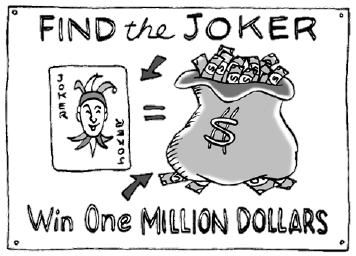### Home > INT3 > Chapter 8 > Lesson 8.3.2 > Problem8-118

8-118.The Algiers High School booster club is planning a fundraiser to collect money for a new synthetic turf field and stadium lights. The boosters will completely cover the football field with playing cards ($2.5$ inches $× 3.5$ inches) face down. All the cards are aligned in the same direction as the football field (long side of the card along the long side of the field). There will be exactly one joker card placed at random among the cards on the field. The football field is $100$ yards $\times53\frac{1}{3}$ yards.

Contestants pay five dollars for every card they wish to turn over. Whoever finds the joker wins one million dollars.

1. What is the probability that the first player finds the joker by turning over one card?

If $100$ yards $= 300$ feet $= 3600$ inches, then $3600 ÷ 3.5 ≈ 1029$ cards will fit along the length of the field.
How many cards will fit across the width of the field?

2. The playing cards used to cover the field cost $0.99$ per pack ($52$ cards per pack). What is the largest amount of money the boosters can lose in this fundraiser?

How many packs of cards are needed? What is this cost?
If the first player wins, they pay $5$, but win $1,000,000$.

3. What is the maximum amount of money the boosters can make?

In order to get the most money each chance must be purchased, meaning $790,272$ people will play.

4. What is a reasonable expected profit for the booster club? That is, if this fundraiser were done many, many times, what would be the average profit?

Assume that on average half the cards will be sold before there was a winner.

$960,633.98$

5. A state Mucho Millions lottery advertises odds of $176$ million to one. If the state lottery were played like the booster club fundraiser, how many football fields covered with non-joker playing cards would be needed?

$\frac{\text{number of cards needed to meet state lottery odds}}{\text{ number of cards per football field}}=\text{number of fields needed}$